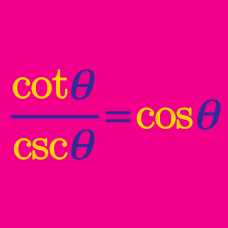Geometry

# Proving Sum and Difference Trigonometric Identities

Which of the following is equal to $\sin(x+y)\sin(x-y)?$

Which of the following is equal to $\displaystyle \frac{\sin x + \sin 3x}{\cos x - \cos 3x}?$

If $x,y,z$ are the internal angles of a triangle, which of the following is equal to the sum $\sin x+\sin y+\sin z?$

Evaluate $\cos^2\theta+\cos^2\left(\theta+\frac{2}{3}\pi\right)+\cos^2\left(\theta-\frac{2}{3}\pi\right).$

If $x,y,z$ are the internal angles of a triangle, which of the following is equal to the sum $\cos^2x+\cos^2y+\cos^2z?$

×